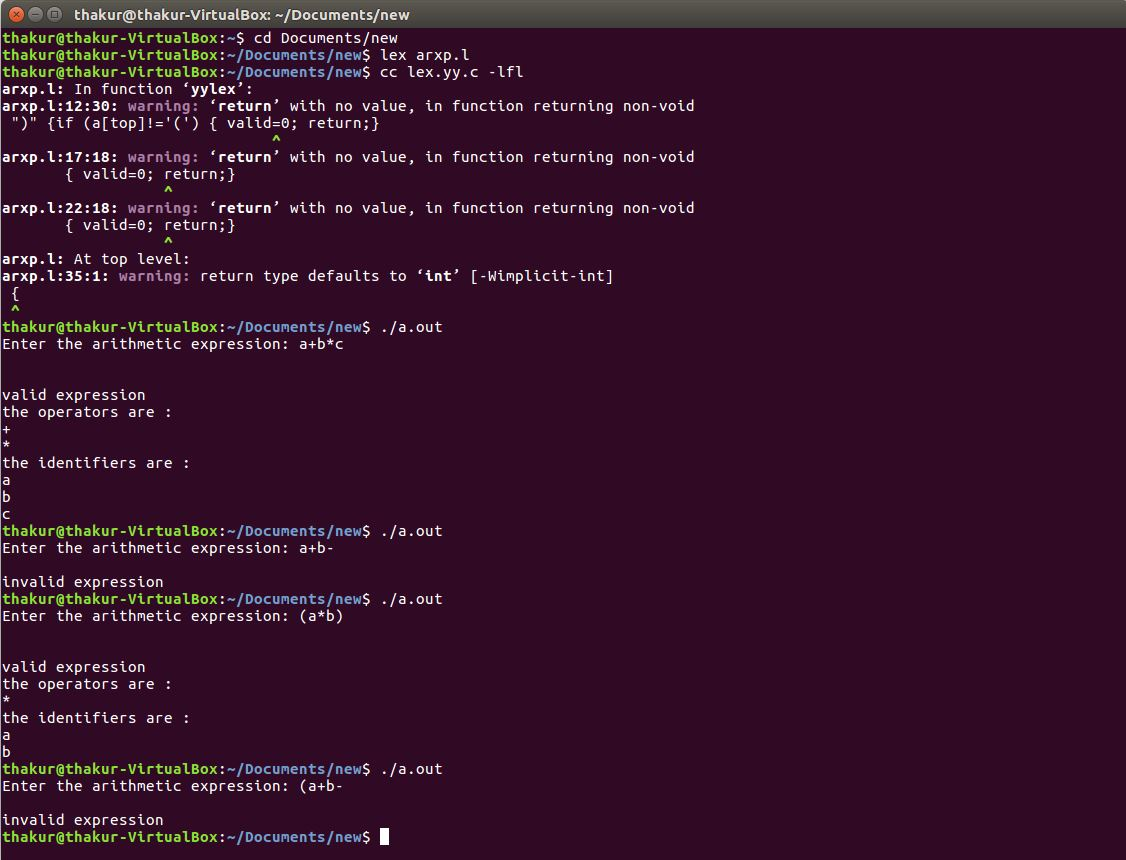Related Articles

# Lex program to recognize valid arithmetic expression and identify the identifiers and operators

• Last Updated : 03 Dec, 2019

Problem: Write a Lex program to recognize valid arithmetic expression and identify the identifiers and operators.

Explanation:
Flex (Fast lexical Analyzer Generator) is a tool/computer program for generating lexical analyzers (scanners or lexers) written by Vern Paxson in C around 1987. Lex reads an input stream specifying the lexical analyzer and outputs source code implementing the lexer in the C programming language. The function yylex() is the main flex function which runs the Rule Section.

Examples:

```Input: a+b*c
Output: valid expression
the operators are :
+
*
the identifiers  are :
a
b
c

Input: a+b-
Output: invalid expression

Input: (a*b)
Output: valid expression
the operators are :
*
the identifiers are :
a
b

Input: (a+b-
Output: invalid expression ```

Implementation:

 `/* Lex program to recognize valid arithmetic expression``        ``and identify the identifiers and operators */``%{ ``#include  ``#include  ``    ``int` `operators_count = 0, operands_count = 0, valid = 1, top = -1, l = 0, j = 0; ``    ``char` `operands, operators, stack; ``%} ``%%``"("` `{ ``    ``top++; ``    ``stack[top] = ``'('``; ``} ``"{"` `{``    ``top++; ``    ``stack[top] = ``'{'``; ``} ``"["` `{ ``    ``top++; ``    ``stack[top] = ``'['``; ``} ``")"` `{ ``    ``if` `(stack[top] != ``'('``) { ``        ``valid = 0;     ``    ``} ``    ``else` `if``(operands_count>0 && (operands_count-operators_count)!=1){``        ``valid=0;``    ``}``    ``else``{``        ``top--;``        ``operands_count=1;``        ``operators_count=0;``    ``} ``} ``"}"` `{ ``    ``if` `(stack[top] != ``'{'``) { ``        ``valid = 0;     ``    ``} ``    ``else` `if``(operands_count>0 && (operands_count-operators_count)!=1){``        ``valid=0;``    ``}``    ``else``{``        ``top--;``        ``operands_count=1;``        ``operators_count=0;``    ``} ``} ``"]"` `{ ``    ``if` `(stack[top] != ``'['``) { ``        ``valid = 0; ``    ``} ``    ``else` `if``(operands_count>0 && (operands_count-operators_count)!=1){``        ``valid=0;``    ``}``    ``else``{``        ``top--;``        ``operands_count=1;``        ``operators_count=0;``    ``} ``         ` `} ``"+"``|``"-"``|``"*"``|``"/"` `{ ``    ``operators_count++; ``    ``strcpy``(operators[l], yytext); ``    ``l++; ``} ``[0-9]+|[a-zA-Z][a-zA-Z0-9_]* { ``    ``operands_count++; ``    ``strcpy``(operands[j], yytext); ``    ``j++; ``} ``%% `` ` ` ` `int` `yywrap() ``{ ``    ``return` `1; ``} ``int` `main() ``{ ``    ``int` `k; ``    ``printf``(``"Enter the arithmetic expression: "``); ``    ``yylex(); `` ` `    ``if` `(valid == 1 && top == -1) { ``        ``printf``(``"\nValid Expression\n"``); ``    ``} ``    ``else``        ``printf``(``"\nInvalid Expression\n"``); `` ` `    ``return` `0;``} `

Output:Attention reader! Don’t stop learning now. Get hold of all the important CS Theory concepts for SDE interviews with the CS Theory Course at a student-friendly price and become industry ready.

My Personal Notes arrow_drop_up